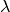# Steps for Detecting Bistability¶

The following are some simple steps to follow for detecting bistability using CRNT4SBML:

1. Construct an SBML file following the guidelines provided in CellDesigner Walkthrough.
2. Check if the conditions for the Deficiency Zero or One Theorems are satisfied using the approach outlined in Low Deficiency Approach.
3. If the Deficiency Zero or One Theorems are not satisfied, then use crnt4sbml.Cgraph.get_dim_equilibrium_manifold() to find, the number of mass conservation relationships.
4. Ifis greater than zero one can use the details described in Mass Conservation Approach Walkthrough to conduct further analysis of a uniterminal network.
5. Ifis greater than zero one can use the details described in General Approach Walkthrough to conduct further analysis of any network.
6. Ifis zero and there is a boundary species present in the SBML file then one can use the details described in Semi-diffusive Approach Walkthrough to conduct further analysis of the network.